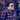# Ruby Enumerable, Part 2

August 06, 2014

The Enumerable module can be used for all of your Ruby classes as long as they implement their own each method. A good example of this are the Active Record Collection Objects. The Rails team has each defined in ActiveRecord.

### each

`#each` can be implemented on any object and what it does is it iterates through a collection and performs the block on each element of the collection.

``````User.all.each { |user| puts user.email }
charlesdmassry@gmail.com
wombat@example.com``````

For every method in Enumerable, it turns to `#each` for every method and uses each to perform the method.

### each_cons

`#each_cons` returns a group of arrays of consecutive indices with the length specified by the number in the parameter. It then performs the block operation on each array.

``````[1, 2, 3, 4].each_cons(2) { |a| p a }
[1, 2]
[2, 3]
[3, 4]

{ name: 4, age: 5, ssn: 6 }.each_cons(2) { |a| p a }
[[:name, 4], [:age, 5]]
[[:age, 5], [:ssn, 6]]``````

One collection is turned into an array that contains the number of objects in an argument and performs the operation of the block on each array.

### each_entry

`#each_entry` you can just use `#each` for similar results.

### each_slice

`#each_slice` takes an argument and similar to `#each_cons`, performs an operation on a new sub-array. This method however, doesn’t make consecutive arrays but makes slices of arrays.

``````[1, 2, 3, 4].each_cons(2) { |a| p a }
[1, 2]
[3, 4]

{ name: 4, age: 5, ssn: 6 }.each_cons(2) { |a| p a }
[[:name, 4], [:age, 5]]
[[:ssn, 6]]``````

Here you can see it can return an uneven output for mismatched elements.

### eachwithindex

`#each_with_index` performs each on the collection and also keeps track of the current index.

``````user = []
%w(name age ssn).each_with_index do |element, index|
user << element * index
end
["", "age", "ssnssn"]``````

It can be used for a lot more complicated operations that this and will make your code a lot nicer that this.

``````user = []
count = 0
%w(name age ssn).each do |element|
user << element
count += 1
end``````

### eachwithobject

`#each_with_object` works by taking a block and a parameter and performing the block operation on the current element and the parameter.

``````(1..3).each_with_object([]) { |a, i| p a, i }
1
[]
2
[]
3
[]``````

This method can be used almost identically to `#each`.

``````numbers = [1,2,6]
(1..3).each_with_object(numbers) { |a, i| i << a } #=> [1, 2, 6, 1, 2, 3]``````

The second element in the block argument here is the parameter.

### entries

`#entries` can be used as an alias for `#to_a`. In some instances, it reads a little bit better than `#to_a`.

### find

`#find` returns the first entry where the block conditions are true. It is aliased to detect.

``[1,2,5,7,6].find { |a| a > 5 } #=> 7``

As you can see, `#find` returns an a number for the first one it finds, for this example it is 7 and not 6 because of the ordered array, so it breaks out. Generally, it would be a good idea to sort this array first if you are using the find method.

### find_all

`#find_all` is similar to `#find` except it returns an array where the given conditions are true.

``[1,2,5,7,6].find_all { |a| a > 5 } #=> [7, 6]``

### find_index

`#find_index` returns the index of `#find`.

``[1,2,5,7,6].find_index { |a| a > 5 } #=> 3``

Here it would find the number 7 and see that it is at index 3 and return that number. Stay tuned for more.Written by Charlie Massry who lives and works in New York building useful things. You should follow him on Twitter